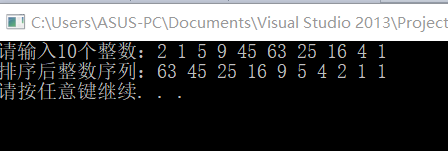2021-06-18 16:17

# 有大佬会这个题吗 C语言的题

• 写回答
• 好问题 提建议
• 关注问题
• 收藏
• 邀请回答

#### 1条回答默认 最新

•抬头见山 2021-06-18 16:31
已采纳
``````#include <iostream>
using namespace std;

void InsertSort(int a[], int n)
{
for (int i = 1; i<n; i++) {
if (a[i] < a[i - 1]) {               //若第i个元素大于i-1元素，直接插入。小于的话，移动有序表后插入
int j = i - 1;
int x = a[i];        //复制为哨兵，即存储待排序元素
a[i] = a[i - 1];           //先后移一个元素
while (x < a[j]) {  //查找在有序表的插入位置
a[j + 1] = a[j];
j--;         //元素后移
}
a[j + 1] = x;      //插入到正确位置
}
}
}

int main() {
int a;
cout << "请输入10个整数：";
for (int k = 0; k < 10; k++)
cin >> a[k];
InsertSort(a, 10);
cout << "排序后整数序列：";
for (int j = 9; j>=0; j--) {
cout << a[j] << " ";
}
cout << endl;
system("pause");
return 0;
}
``````已采纳该答案
评论
解决 1 无用
打赏 举报